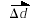### Units and Standards

#### There are 4 fundamental scientific measurements:

• Mass
• Length
• Time
• Electric Charge
The following summarizes their basic SI (International Metric System) units:

 Fundamental Quantity Mass Length, distance, displacement Time Charge SI Unit kg (kilogram) m (metre) s (second) C (coulomb) Symbol m Δd t Q

#### Scalar vs. Vector Quantities

• Scalar quantities express only a quantity and a unit
• Vector quantities express a quantity, a unit and a direction

Examples:

 Quantity Symbol Type Example distance Δd scalar Δd = 10 m displacementvector= 10 m [E]